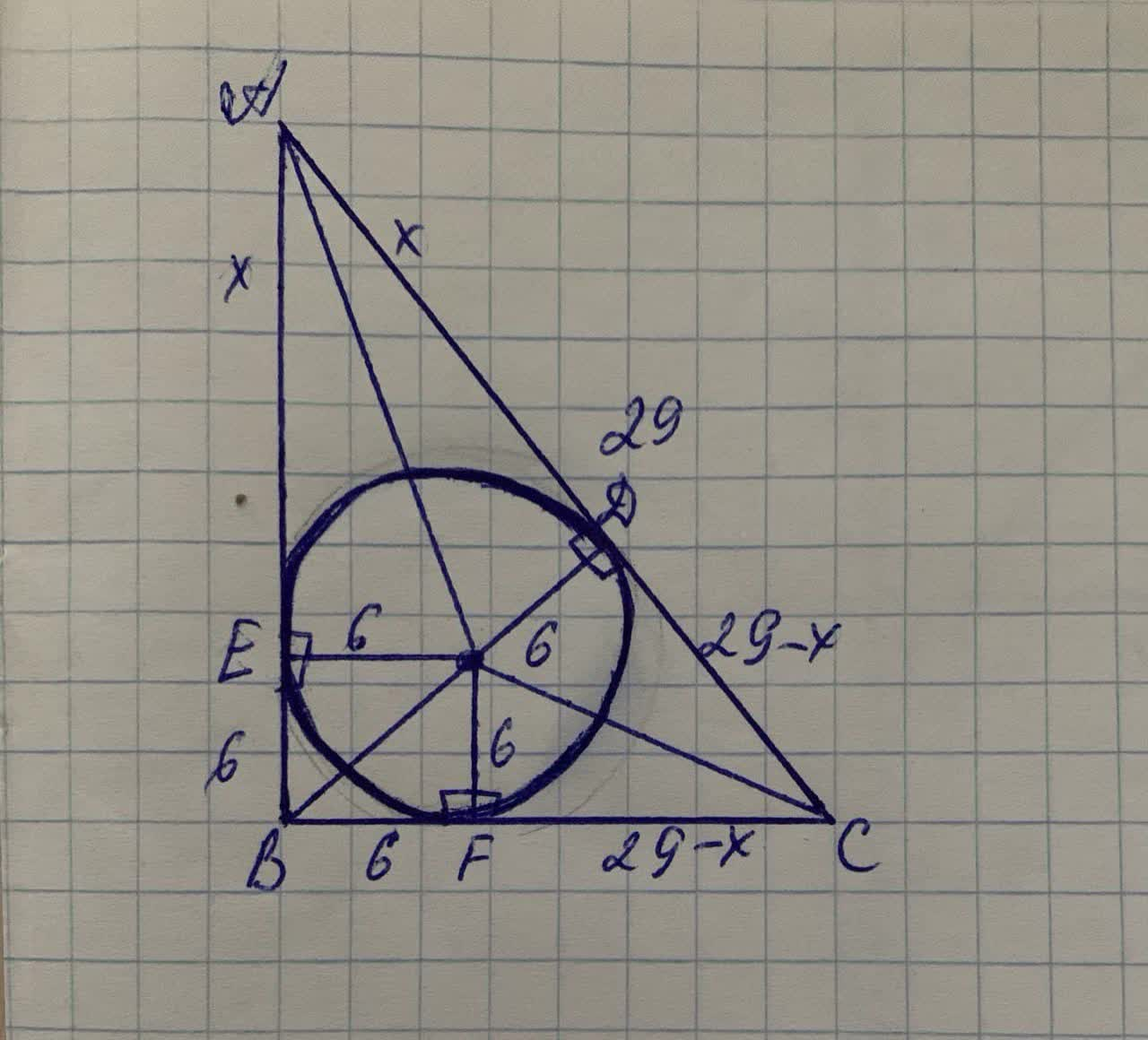A circle is inscribed in a right triangle. The lengthLorenzolaji 2021-11-25 Answered
A circle is inscribed in a right triangle. The length of the radius of the circle is 6 cm, and the length of the hypotenuse is 29 cm. Find the lengths of the two segments of the hypotenuse that are determined by the point of tangency.

• Questions are typically answered in as fast as 30 minutes

Solve your problem for the price of one coffee

• Math expert for every subject
• Pay only if we can solve itSusan Yang
Step 1
Concept:
Similar to circle sphere is a two dimensional space where the set of points that are at the same distance r from a given point in a three dimensional space. In analytical geometry with a center and radius is the locus of all points is called sphere
Step 2
Given:
A circle is inscribed in a right triangle. The length of the radius of the circle is 6 cm, and the length of the hypotenuse is 29 cm.
thus the given condition is can be drawn as follows,The objective is to find the lengths of the two segments of the hypotenuse that are determined by the point of the tangency
Step 3
Using Pythagorean theorem,
$$\displaystyle{A}{C}^{{2}}={A}{B}^{{2}}+{B}{C}^{{2}}$$
$$\displaystyle{A}{C}^{{2}}={\left({A}{E}+{E}{B}\right)}^{{2}}+{\left({B}{F}+{F}{C}\right)}^{{2}}$$
$$\displaystyle{29}^{{2}}={\left({X}+{6}\right)}^{{2}}+{\left({6}+{29}-{X}\right)}^{{2}}$$
$$\displaystyle{29}^{{2}}={\left({X}+{6}\right)}^{{2}}+{\left({35}-{X}\right)}^{{2}}$$
$$\displaystyle{841}={2}{X}^{{2}}-{58}{X}+{1261}$$
Step 4
On solving further
$$\displaystyle{2}{x}^{{2}}-{58}{x}+{420}={0}$$
$$\displaystyle{x}{\left({x}-{14}\right)}-{15}{x}+{210}={0}$$
$$\displaystyle{\left({x}-{15}\right)}{\left({x}-{14}\right)}={0}$$
Here
$$\displaystyle{x}={15}$$ or $$\displaystyle{x}={14}$$
Therefore, the lengths of the two segments are as follows, 15 and 14 cm
Not exactly what you’re looking for?nick1337

Step 1
Given: The length of the radius of the circle is 6 cm and the length of the hypotenuse is 29 cm.
To find: The lengths of the two segments of the hypotenuse that are determined by the point of tangency.
Step 2
Let A, B, and C be vertices of the triangle.
And $$\angle ABC=90^{\circ}$$
Then AC=29 cm is the hypotenuse of the triangle.
AB, BC, and CA are the sides of the triangle.
Also, AB, BC, and CA are the tangents of the circle at R, Q and P respectively.
The tangent segments to a circle from an external point are
congruent.
ROBQ is a square.
So, radiii $$RO=QO=BO=OP=6\ cm$$.
And $$BQ=BR=6\ cm$$
So, let $$\overline{AR}=\overline{AP}=x\ and\ \vec{PC}=\overline{QC}=y$$
Because $$\triangle ABC$$ is right triangle, $$AC^{2}=AB^{2}+BC^{2}$$
$$(x+y)^{2}=(x+6)^{2}+(y+6)^{2}$$
$$x^{2}+y^{2}+2xy=x^{2}+12x+36+y^{2}+12y+36$$
$$2xy=12x+36+12y+36$$
$$xy=6x+18+6y+18$$
$$xy=6(x+y)+36$$
$$=6(29)+36$$
$$=174+36$$
$$=210$$
Step 3
$$x+y=29$$
$$y=29-x$$
$$x(29-x)=210\ [xy=210]$$
$$29-x^{2}=210$$
$$x^{2}-29x+210=0$$
$$x^{2}-15x-14x+210=0$$
$$x(x-15)-14(x-15)=0$$
$$(x-14)(x-15)=0$$
$$x=14\ and\ 15$$
If $$x=14\ then\ y=15$$
If $$x=15\ then\ y=14.$$